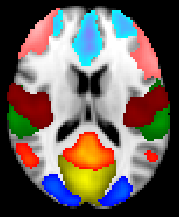## Reproducible Resting State Network (RSN) detection with RAICAR_N

### Abstract

Independent component analysis (ICA) is a popular analysis technique for neuroimaging data. Spatial ICA (SICA) decomposes the time by space functional MRI (fMRI) matrix into a set of 1-D basis time courses and their associated 3-D spatial maps which are optimized for non-Gaussianity and hence mutual independence.

• When applied to resting state fMRI (rsfMRI), SICA produces several interesting spatial components, the so called resting state networks (RSNs).

• The mixing matrix in ICA is identifiable provided that the number of Gaussian sources in the mixture is at most 1. Unfortunately, the contrast function in ICA depends on the finite observed data and is non-convex with potentially many local minima. This means that each run of ICA will produce potentially different RSN networks for the same data!

• A technique to deal with the run to run variability of ICA was proposed in Yang et al. (2008) in their algorithm RAICAR (Ranking and averaging independent component analysis by reproducibility). The basic idea is to select only those ICA components as "interesting" which show a high run to run "reproducibility".

• We propose a simple enhancement to the original RAICAR algorithm to attach "reproducibility" p-values to each ICA component from RAICAR.

### Main ideas

1. The reproducibility indices calculated in RAICAR differ in magnitude significantly depending on whether:

• (a) Input to RAICAR is generated using multiple ICA runs on the same data or

• (b) Input to RAICAR comes from multiple ICA runs on varying data sets (e.g. multiple between and across subject runs)

2. Obviously, the reproducibility indices are much lower in case (b) above since we account for both within subject and between subjects variability in estimating ICs. Note that case (b) is of great interest from a practical point of view since we are often interested in making statements about a group of subjects.

3. Hence it is clear that a cutoff on RAICAR reproducibility values for the purposes of selecting the "highly reproducible" components should be data dependent.

4. We propose to extend the original RAICAR algorithm by using simulation to automatically generate p-values for each reproducible component. This allows for an objective cutoff specification for extracting reproducible components (e.g. reproducible at p < 0.05). We call the resulting algorithm RAICAR_N (N stands for null hypothesis test).

### Key references

1. Pendse GV, Borsook D and Becerra L. A simple and objective method for reproducible resting state network (RSN) detection in fMRI. arXiv:1108.2248v1 [stat.AP], submitted, May, 2011. [pdf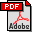] (this work)

2. Rao CR. A Decomposition Theorem for Vector Variables with a Linear Structure. The Annals of Mathematical Statistics, 40(5):1845-1849, 1969.

3. Theis FJ. A New Concept for Separability Problems in Blind Source Separation. Neural Computation, 16:1827-1850, 2004.

4. Yang Z, LaConte S, Weng X and Hu X. Ranking and Averaging Independent Component Analysis by Reproducibility (RAICAR). Human Brain Mapping, 29:711-725, 2008.

5. Beckmann CF, DeLuca M, Devlin JT and Smith SM. Investigations into resting-state connectivity using independent component analysis. Philos. Trans. R. Soc. Lond. B. (Biol Sci), 360(1457):1001-1013, 2005.

6. Smith SM, Fox PT, Miller KL, Glahn DC, Fox PM, Mackay CE, Filippini N, Watkins KE, Toro R, Laird AR, and Beckmann CF. Correspondence of the brain's functional architecture during activation and rest. Proc. Natl. Acad. Sci. U.S.A., 106(31):13040-13045, 2009.

7. Beckmann CF and Smith SM. Probabilistic independent component analysis for functional magnetic resonance imaging. IEEE Transactions on Medical Imaging, 23:137-152, 2004.

### How does RAICAR_N enable the objective selection of "reproducible" ICA components?

1. Suppose an investigator performs group ICA on a set of subjects and sets the number of ICA components = 40. Should all 40 components be reported in a paper? How can the investigator select an "interesting subset" of these 40 components for the purpose of reporting in a paper?

2. Are we really interested in those ICA components that are applicable to a specific run of ICA on a specific set of subjects?

3. Clearly, we are interested in only those ICA components that "generalize" well i.e., are "reproducible" across variations in the data (e.g., across subjects) as well as variations in multiple runs of ICA. A RAICAR analysis enables us to assign reproducibility values to each ICA component.

4. The typical values of normalized reproducibility obtained from RAICAR are dependent on the nature of data.

5. When multiple ICA runs on a single subject are used as inputs to RAICAR, we typically get much higher values of normalized reproducibility compared to the case when combining multi subject ICA runs.

6. The figure below illustrates this effect:

Within subject ICA runs Across subjects ICA runs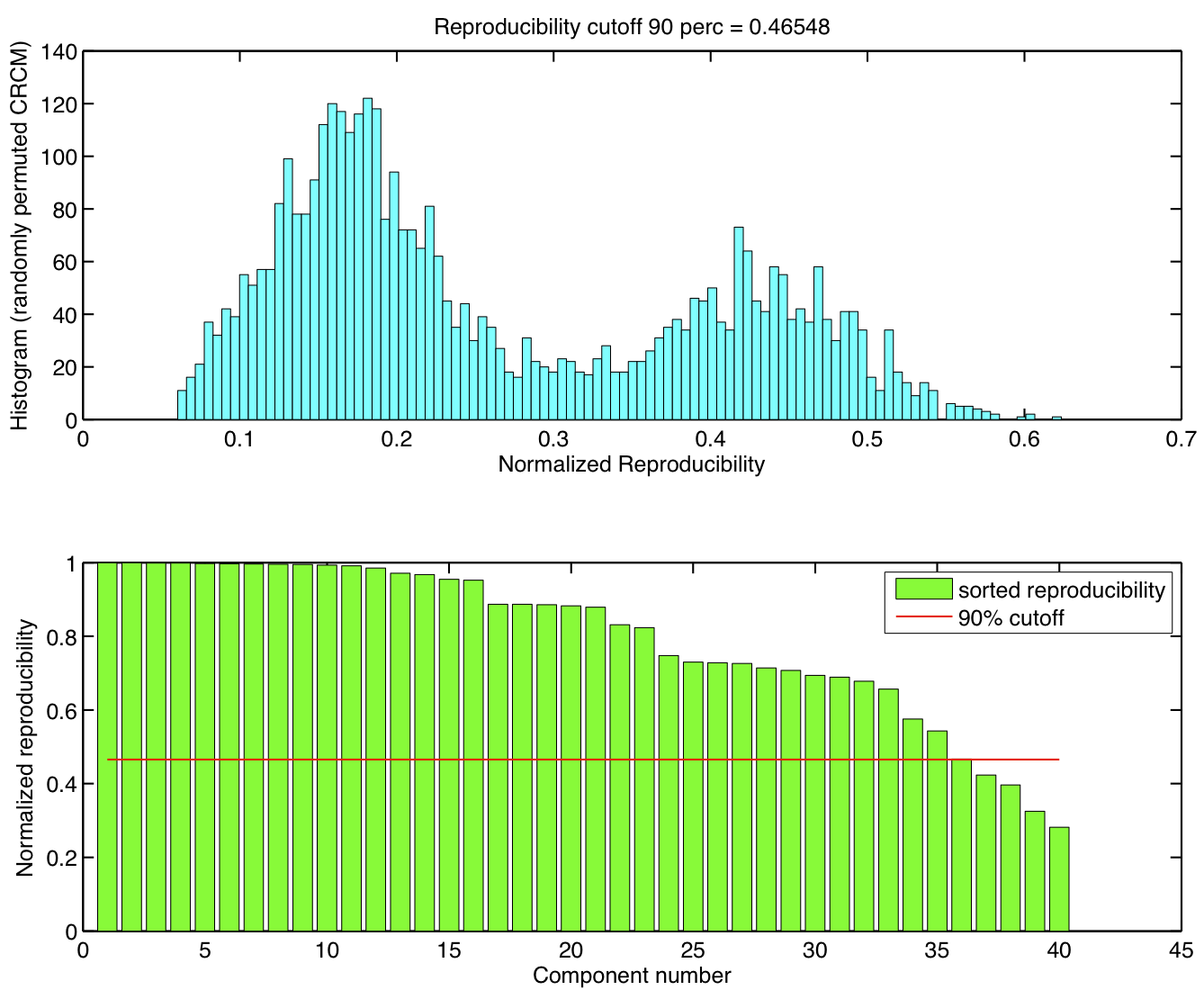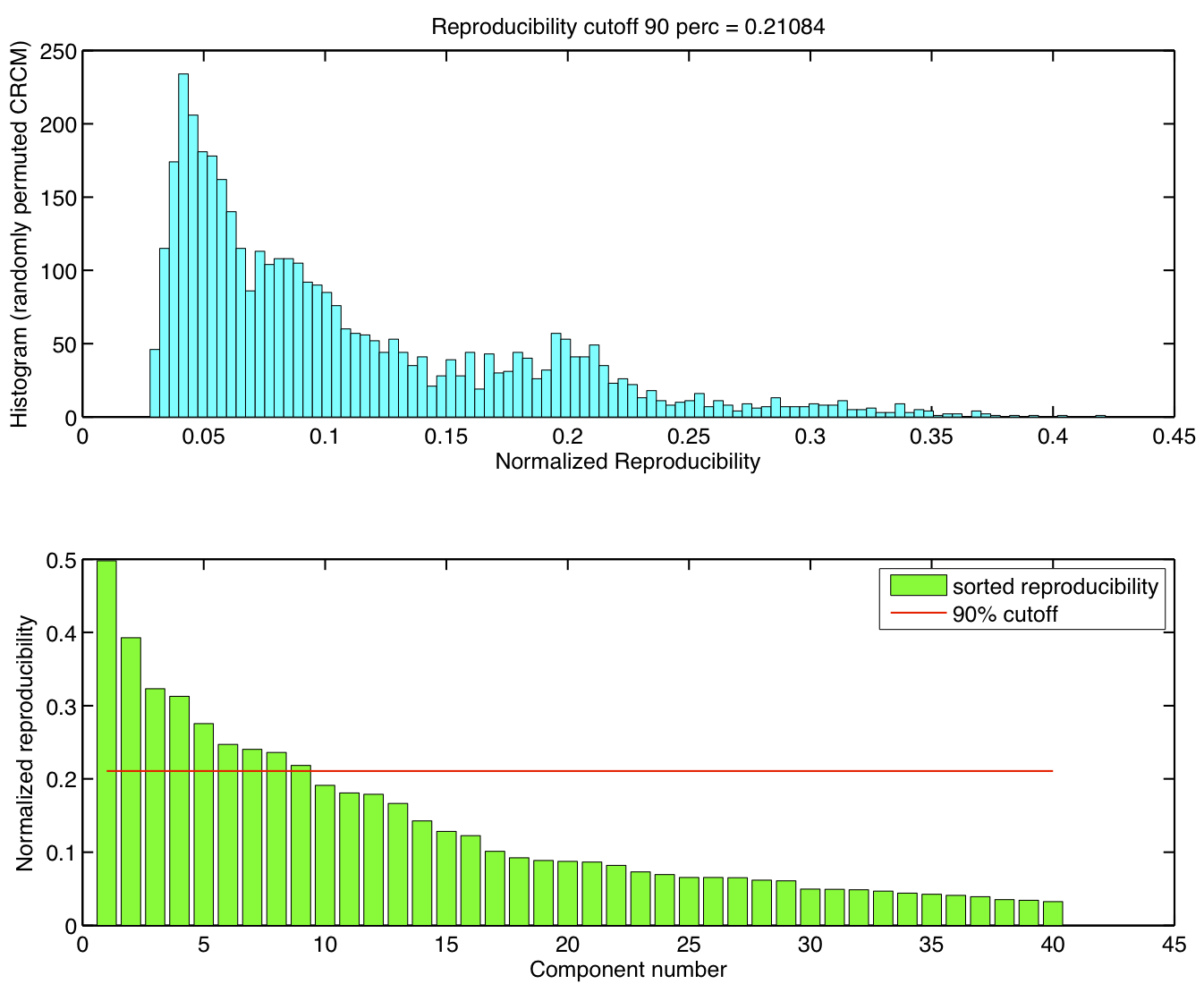7. In RAICAR_N, we are able to compute p-values for each ICA component (see the figure titled RAICAR_N algorithm in the PDF Figures section for a pictorial description of RAICAR_N). Consequently, as shown in the figure below the same and objective p-value cutoffs (e.g. p < 0.05) can be used to identify reproducible components within and across subjects.

Within subject ICA runs Across subjects ICA runs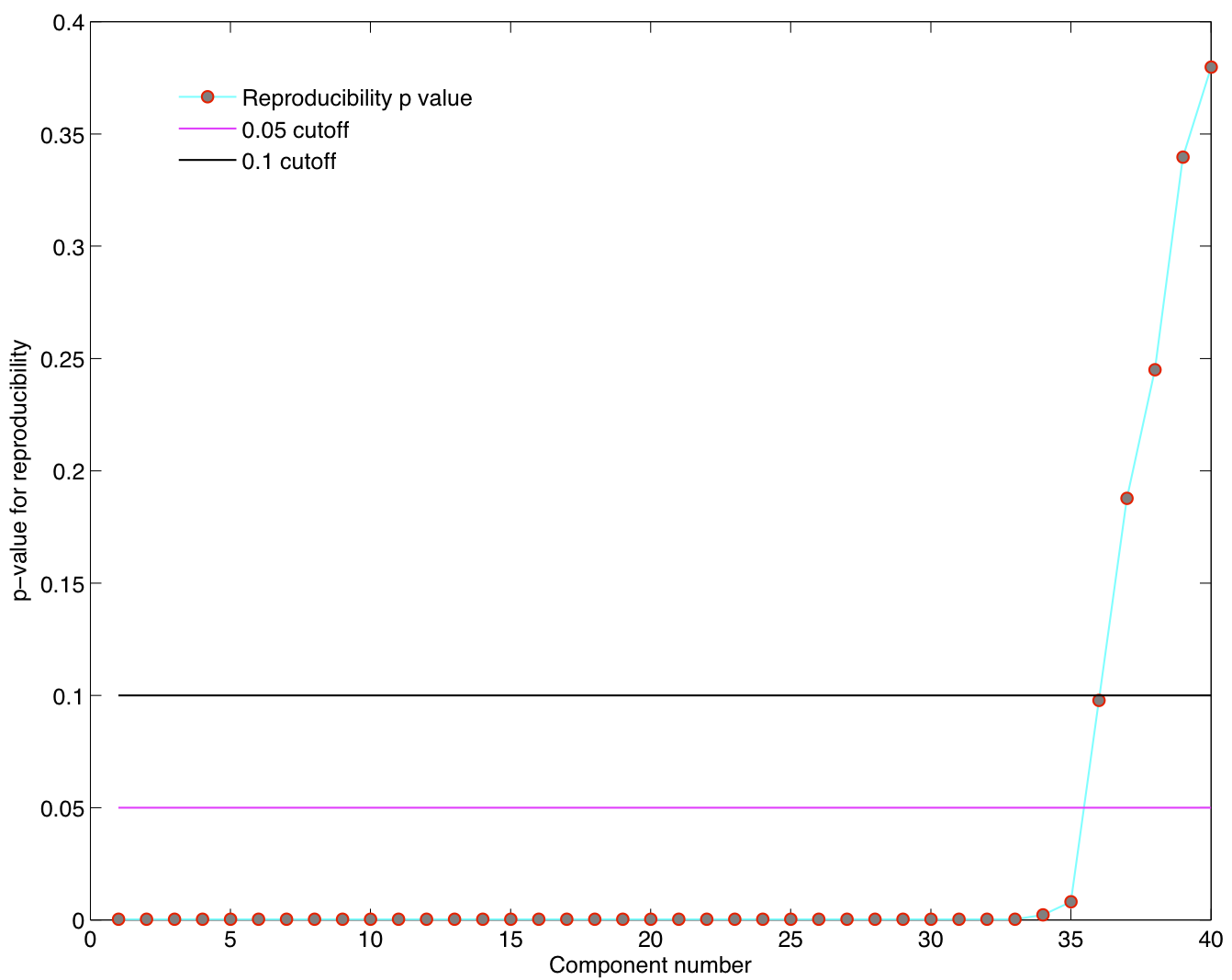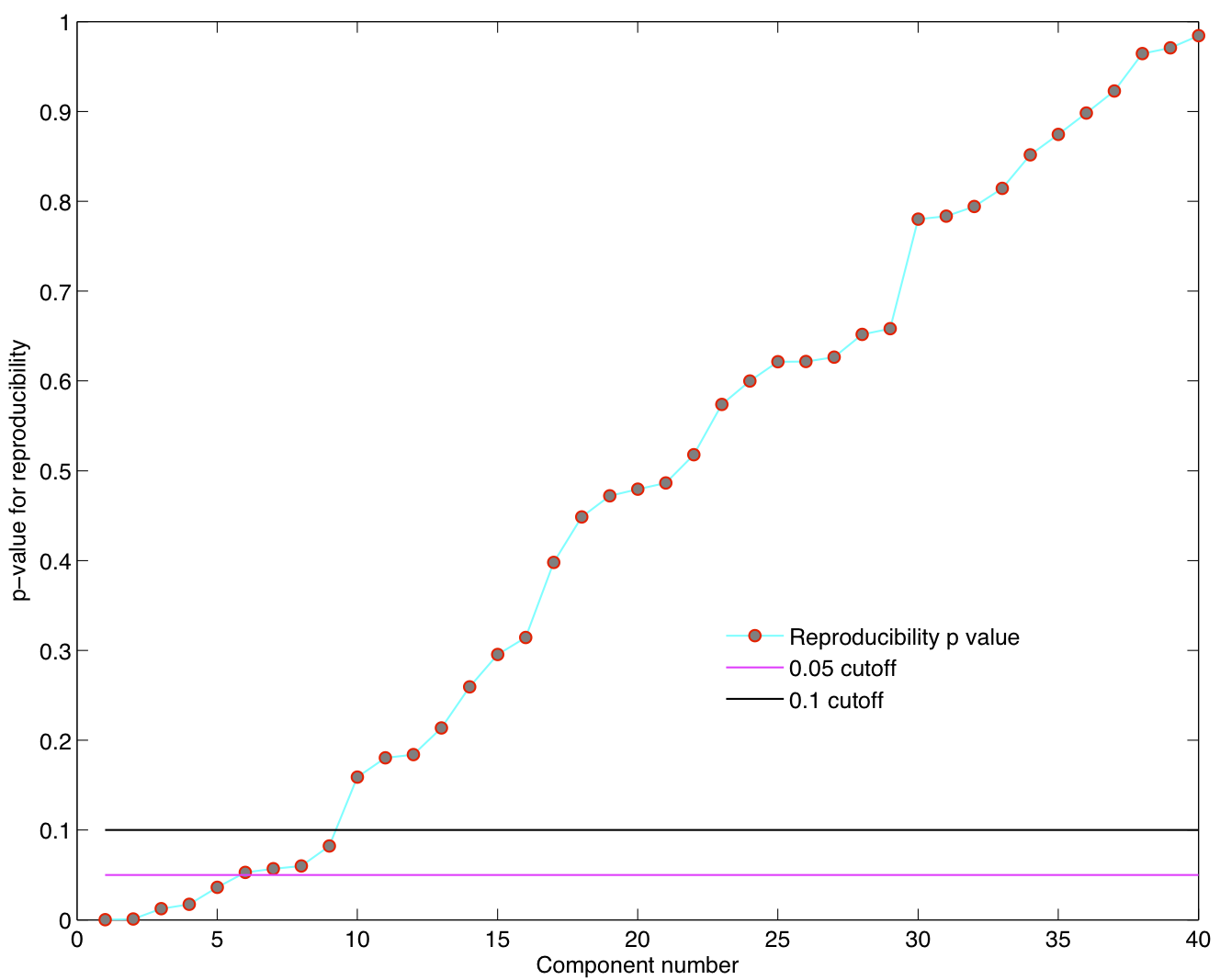### How to choose the number of subjects per group ICA run?

1. Suppose we have a group of N subjects. We randomly select L subjects and form a single group of subjects.

2. We repeat this process K times to get K groups of L subjects each of which is subjected to a group ICA analysis.

3. Given the number of subjects N, how should we choose L and K?

4. The basic idea is to promote dataset diversity in the individual group ICA runs. The figures below explain the choice of L and K in detail.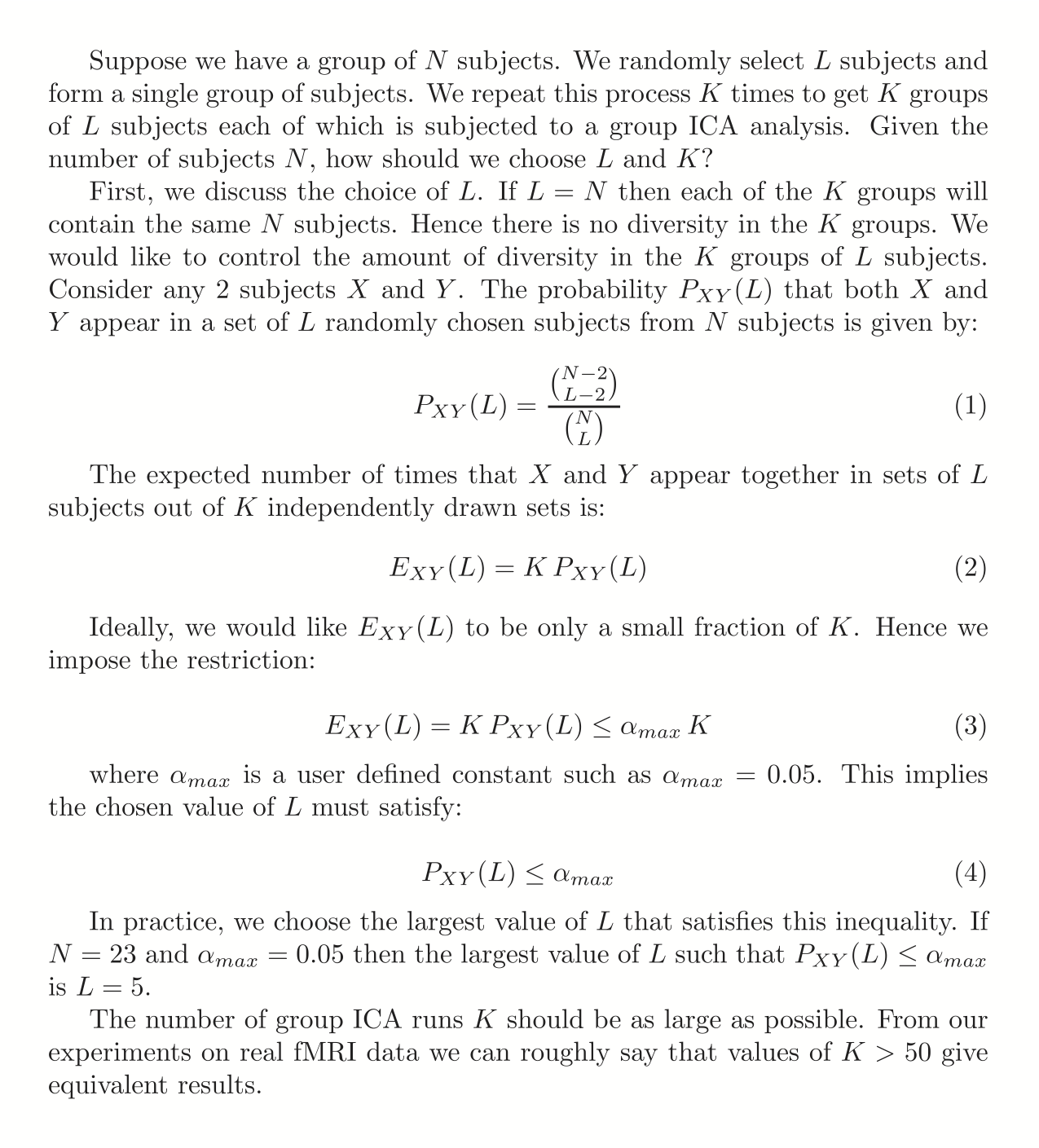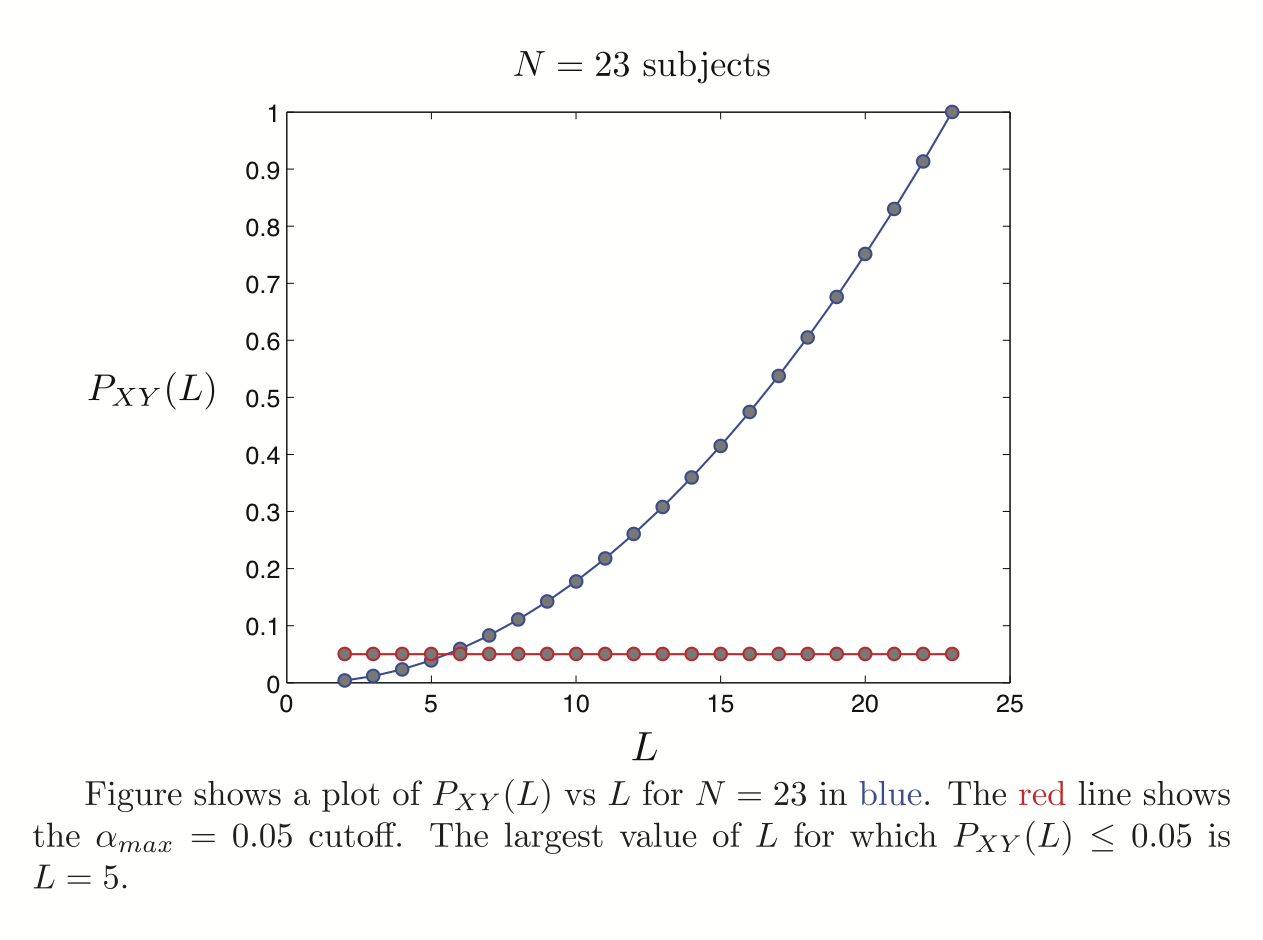### How to display the estimated non-Gaussian spatial structure in ICA maps?

1. The output of a RAICAR_N analysis is a set of spatial ICA maps (either z-transformed maps or raw maps) concatenated into a 4-D volume.

2. We do a voxelwise transformation to Normality using the voxelwise empirical cumulative distribution function.

3. Next, we submit the resulting 4-D volume to a voxelwise group analysis using ordinary least squares. The design matrix for group analysis depends on the question being considered. In our case, the design matrix was simply a single group average design.

4. The resulting t-statistic maps are subjected to Student t, Gamma_pos and Gamma_neg mixture modeling. The logic is that if the original ICA maps are pure Gaussian (i.e., have no interesting non-Gaussian structure) then the result of a group average analysis will be a pure Student t map which will be captured by a single Student t (i.e., the Gamma_pos and Gamma_neg will be driven to 0 class fractions). Hence the null hypothesis will be correctly accounted for. If the Gamma distributions have > 0.5 posterior probability at some voxels then those voxels are displayed in color to indicate the presence of significant non-Gaussian structure over and above the background Student t distribution.

5. Examples of Studen t/Gamma_pos/Gamma_neg mixture modeling are shown below: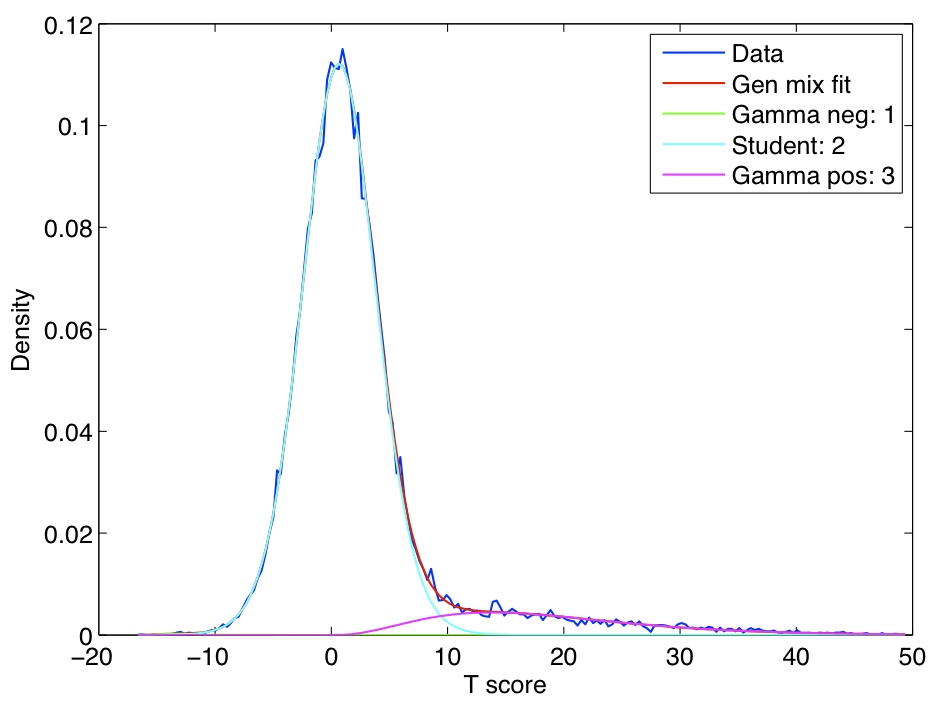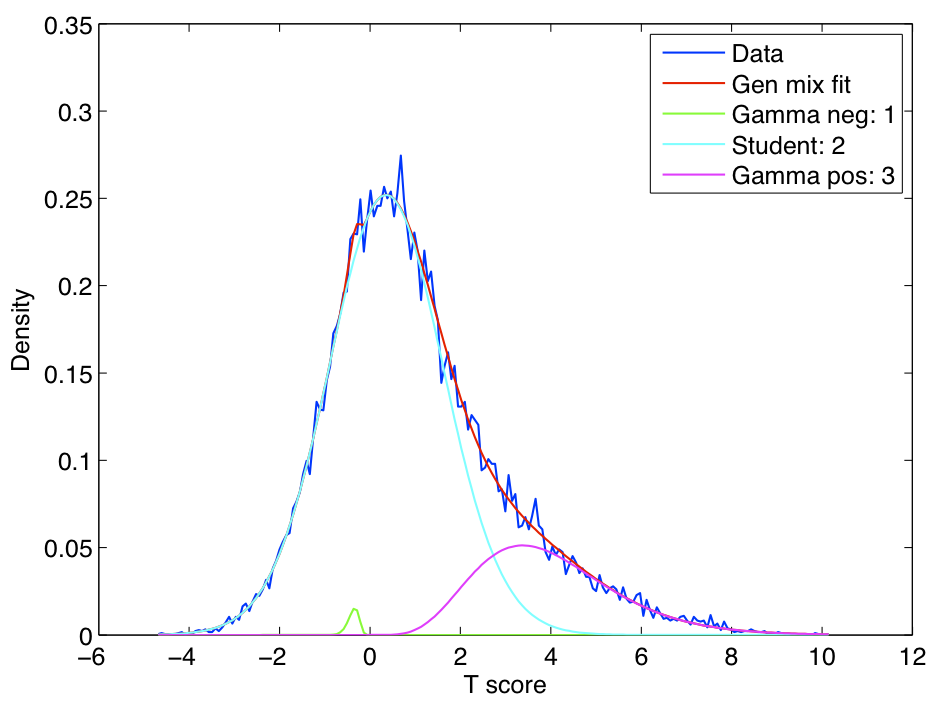### Examples of the application of RAICAR_N to human rsfMRI data in single subject and group mode

rsfMRI data titled: Baltimore (Pekar, J.J./Mostofsky, S.H.; n = 23 [8M/15F]; ages: 20-40; TR = 2.5; # slices = 47; # timepoints = 123) was downloaded from http://www.nitrc.org/. Data was analyzed using tools from the FMRIB software library (FSL, http://www.fmrib.ox.ac.uk/fsl/). Preprocessing steps included motion correction, brain extraction, spatial smoothing with an isotropic Gaussian kernel of 5mm FWHM and 100s high-pass temporal filtering. Spatial ICA was performed using FSL MELODIC in either single subject or multi-subject temporal concatenation mode. In each case, we fixed the number of ICA components at 40. For temporal concatenation mode, single subject data was affinely registered to the MNI 152 brain and subsequently resampled to 4x4x4 resolution (MNI 4x4x4).

#### Multisubject with 1 ICA run per subject

1. ##### Reproducibility p-values
Spatial ICA was run once for each of the 23 subjects in their native space. The resulting set of ICA components across subjects were transformed to MNI 4x4x4 space and were submitted to a RAICAR_N analysis. ICA components were sorted according to their reproducibility and p-values were computed for each ICA component.2. ##### Top 8 components with highest reproducibility compared with "standard" RSN maps found in literature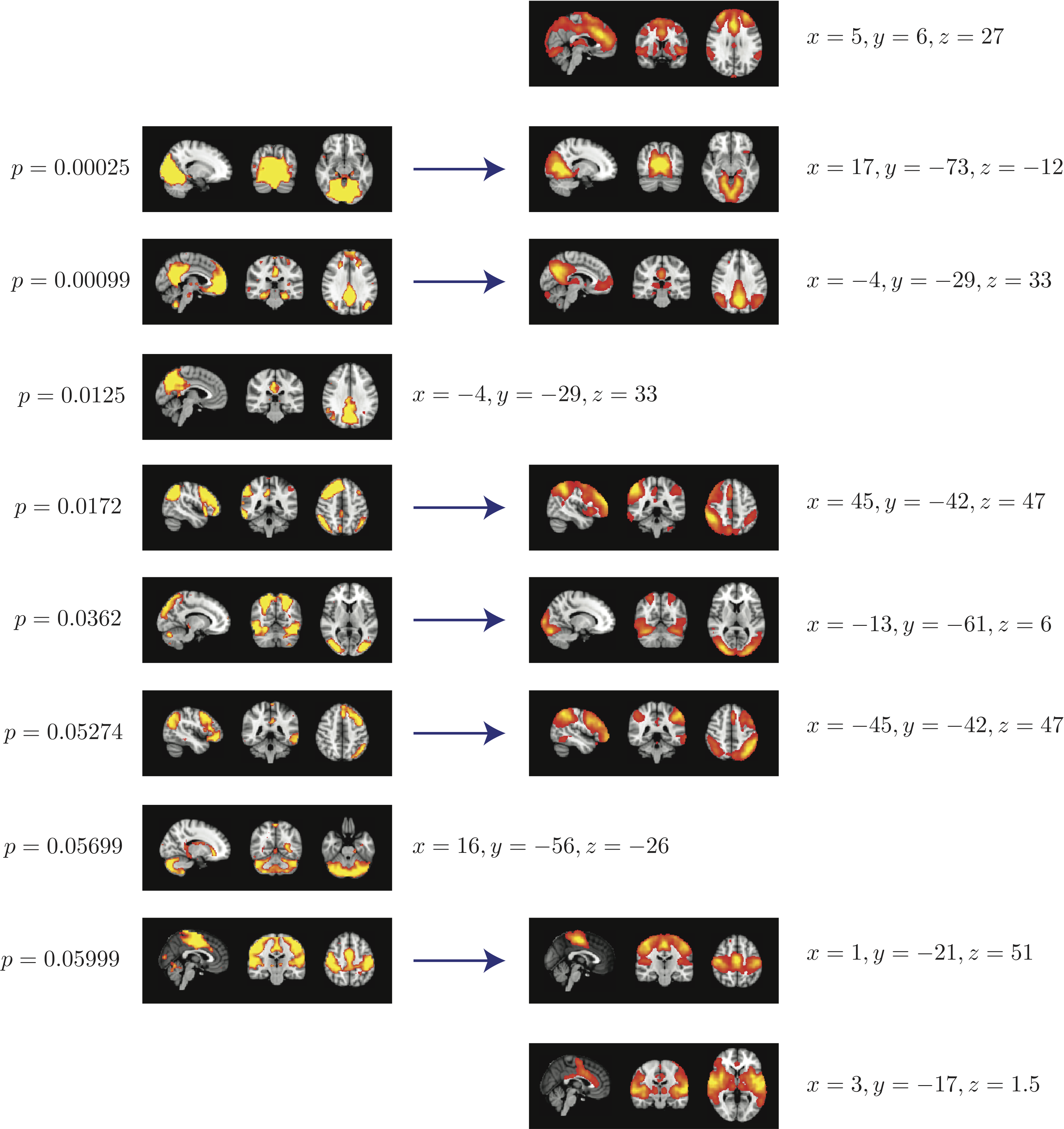3. ##### Summary
When single subject ICA runs are combined across subjects:

• We are able to declare 4 "standard" RSNs as significantly reproducible at a p-value < 0.05.

• There are 2 other "standard" RSNs that achieve a reproducibility p-value between 0.05 and 0.06.

• There are 2 other "non-standard" RSNs that are of interest: one achieves a p-value of 0.0125 and the other achieves a p-value of 0.05699.

#### Random sets of 5 subjects - 50 group ICA runs

1. ##### Reproducibility p-values
5 subjects were drawn at random from the group of 23 subjects and submitted to a temporal concatenation based group ICA. This process was repeated 50 times and the resulting set of 50 group ICA maps were submitted to a RAICAR_N analysis. ICA components were sorted according to their reproducibility and p-values were computed for each ICA component.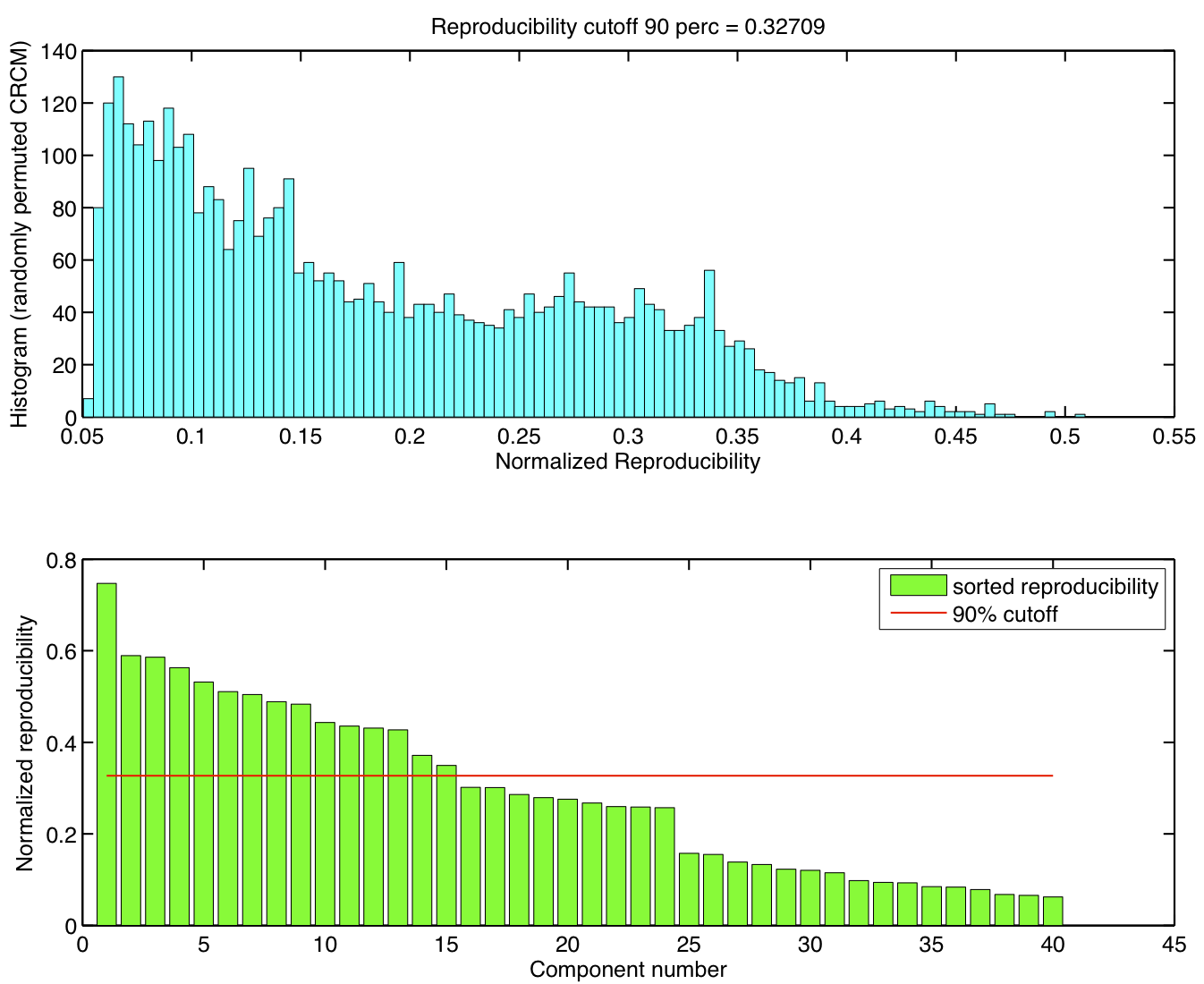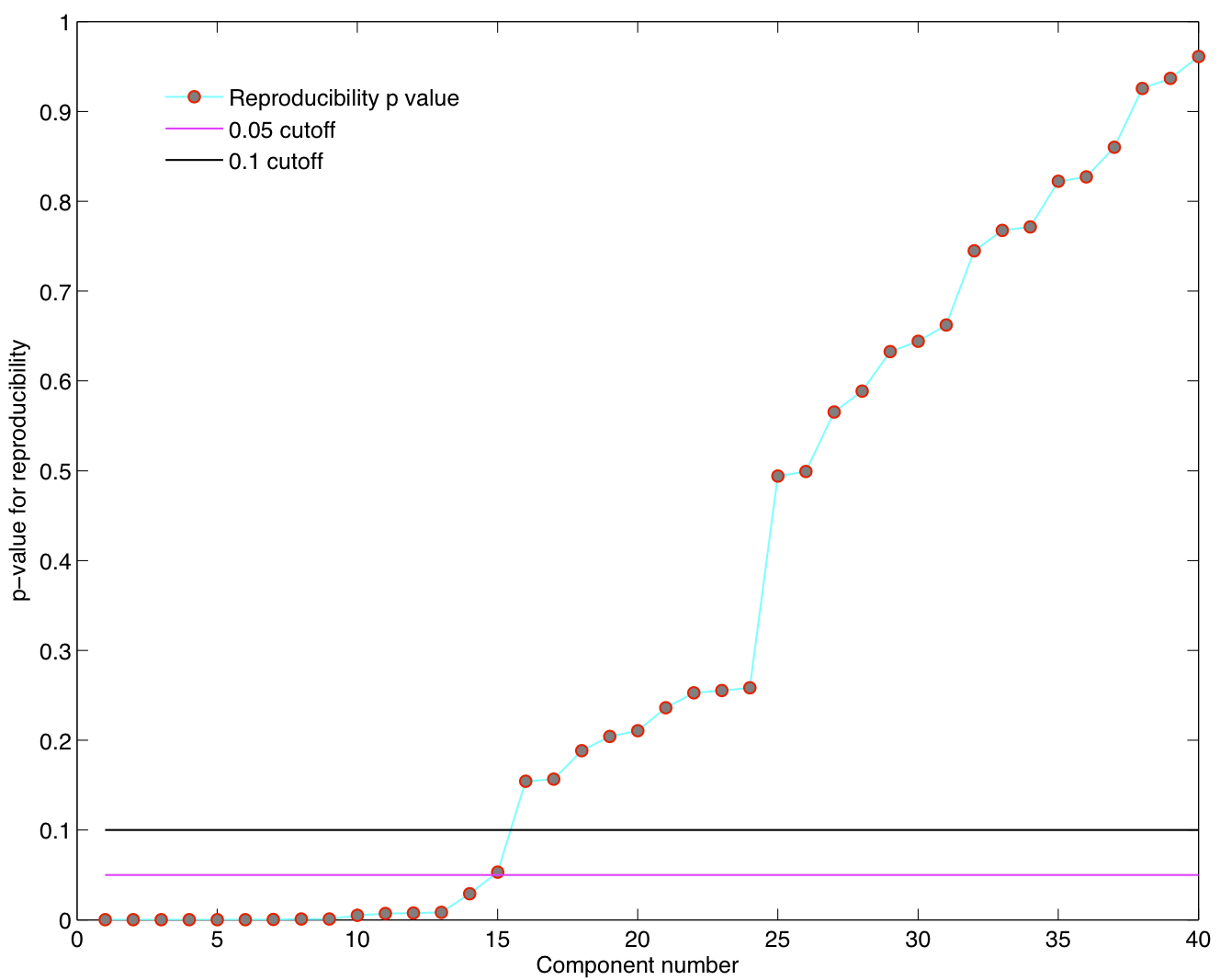2. ##### Top 15 components with highest reproducibility compared with "standard" RSN maps found in literature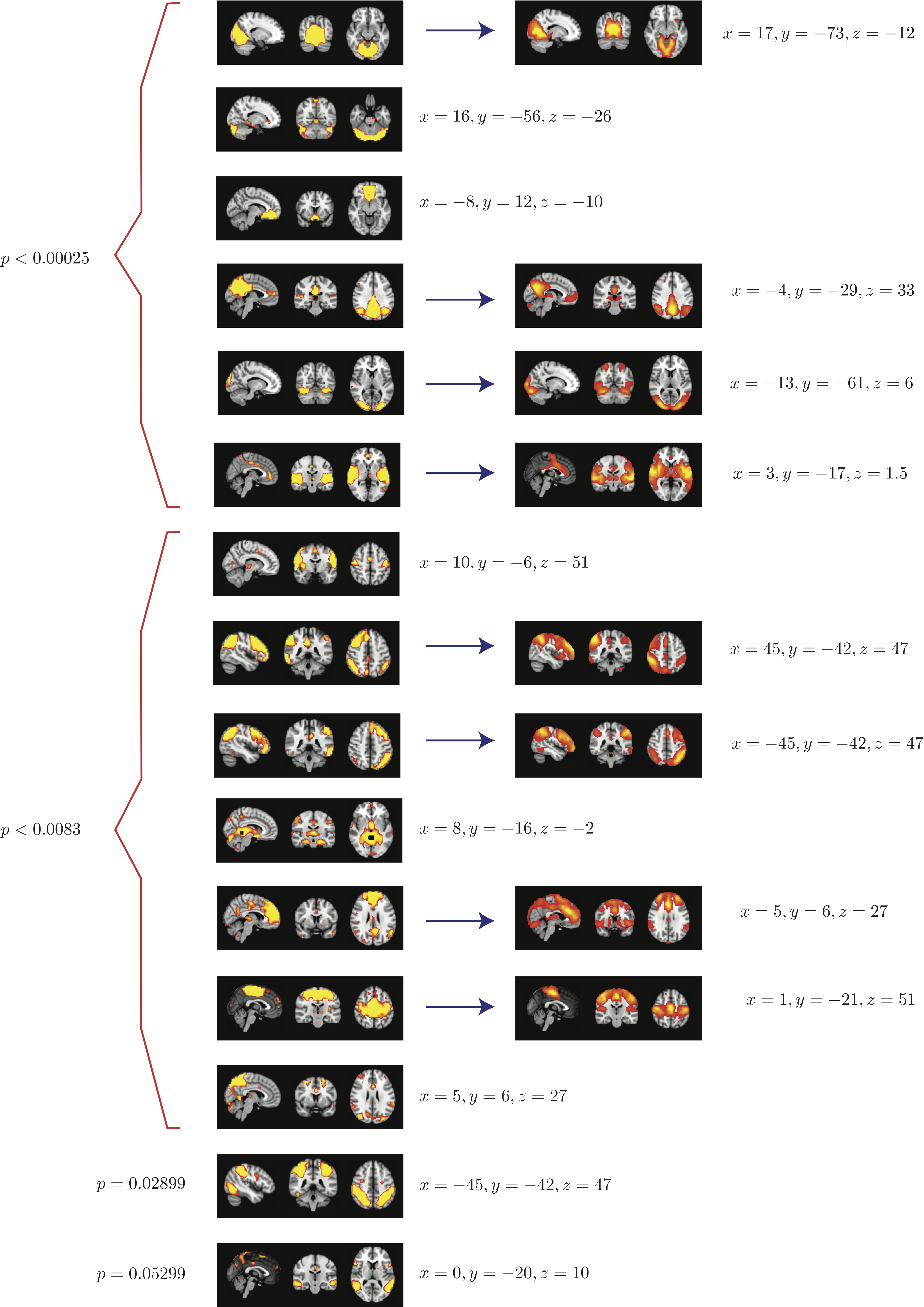3. ##### Summary
When 50 random 5 subject group ICA runs (from a population of 23 subjects) are combined using RAICAR_N:

• We are able to declare 8 "standard" RSNs as significantly reproducible at a p-value < 0.05.

• There are 6 other "non-standard" RSNs that can be declared as significantly reproducible at a p-value < 0.05.

• There is 1 other "non-standard" RSN that achieves a p-value of 0.05299.

#### Random sets of 5 subjects - 100 group ICA runs

1. ##### Reproducibility p-values
5 subjects were drawn at random from the group of 23 subjects and submitted to a temporal concatenation based group ICA. This process was repeated 100 times and the resulting set of 100 group ICA maps were submitted to a RAICAR_N analysis. ICA components were sorted according to their reproducibility and p-values were computed for each ICA component.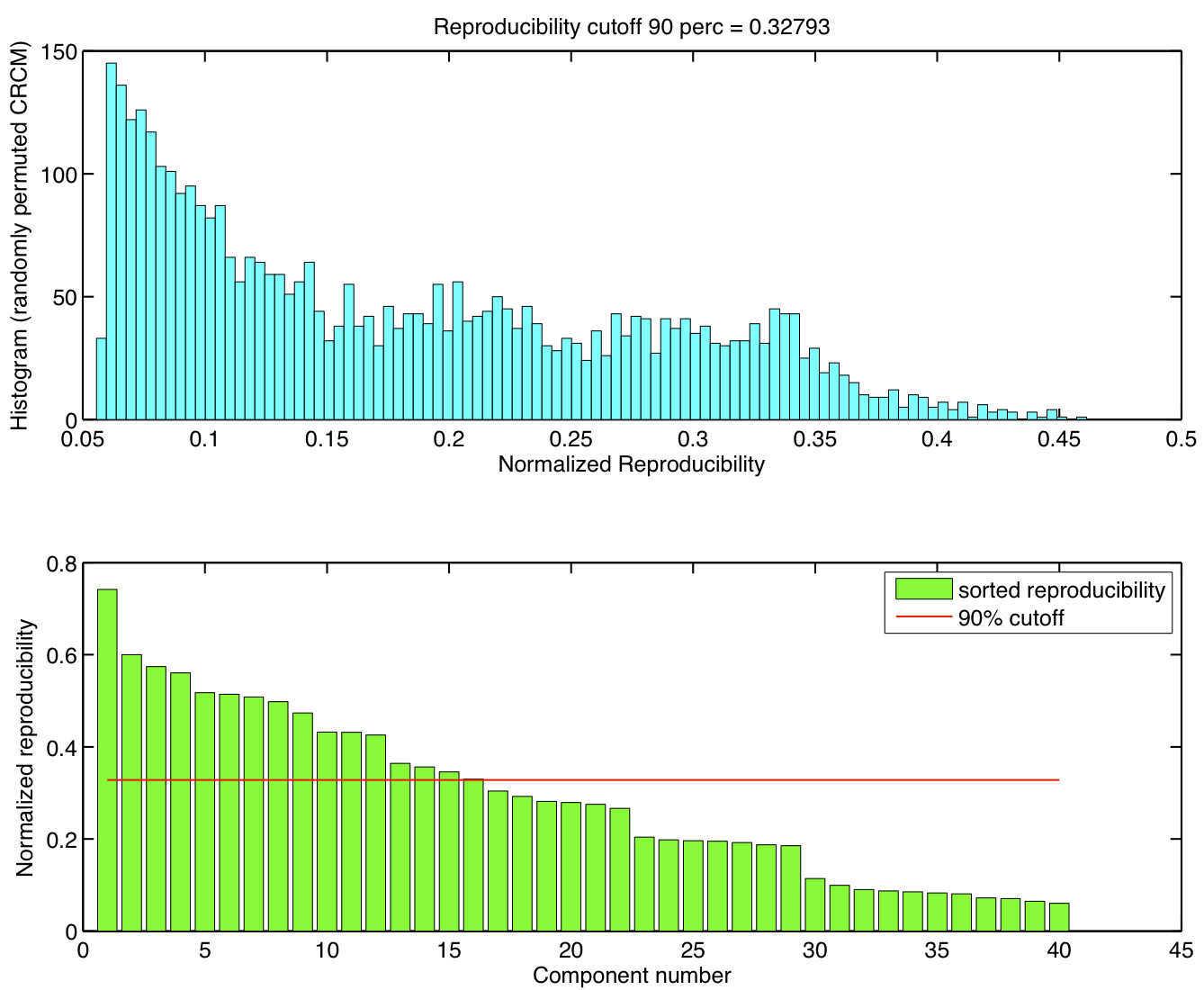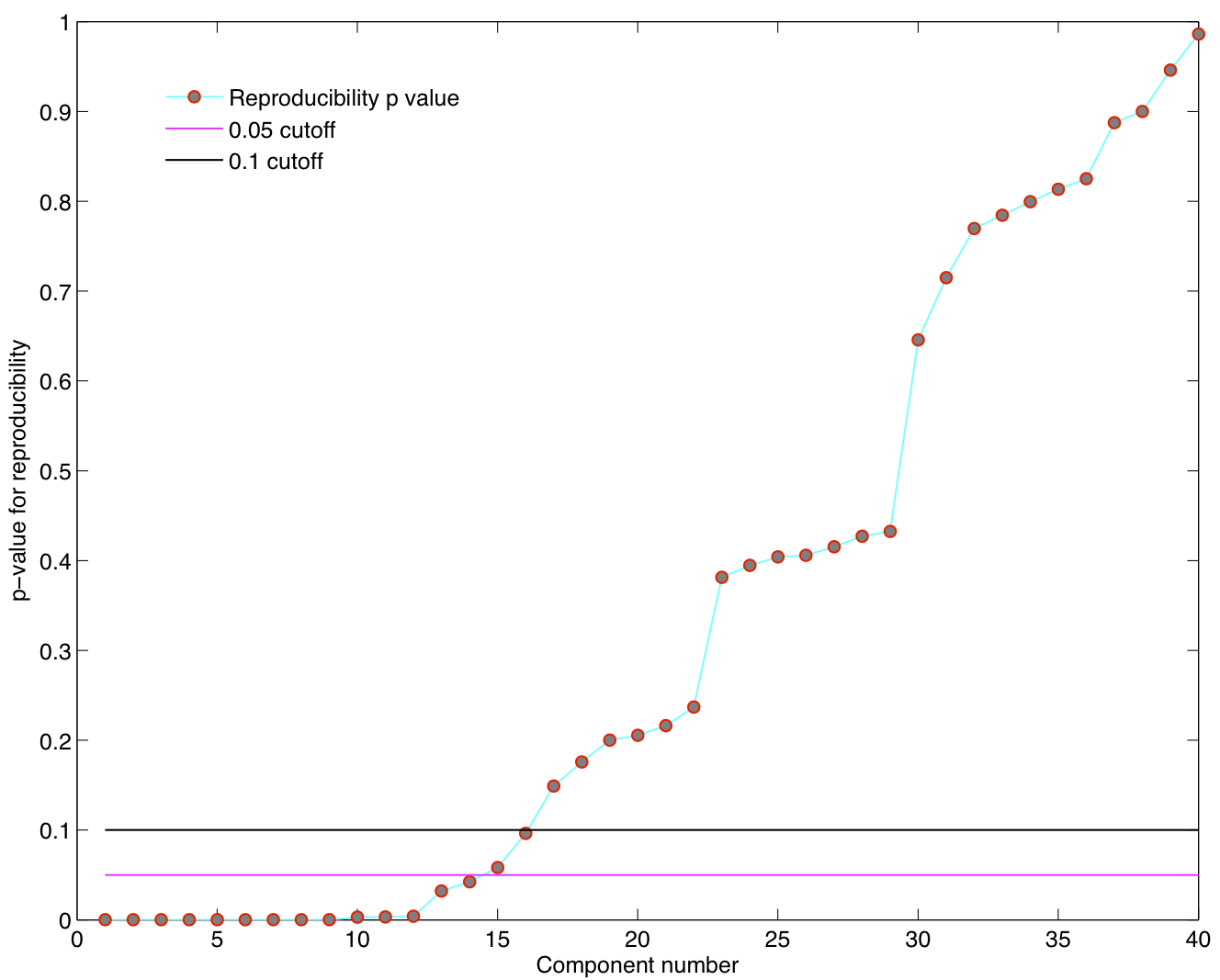2. ##### Top 15 components with highest reproducibility compared with "standard" RSN maps found in literature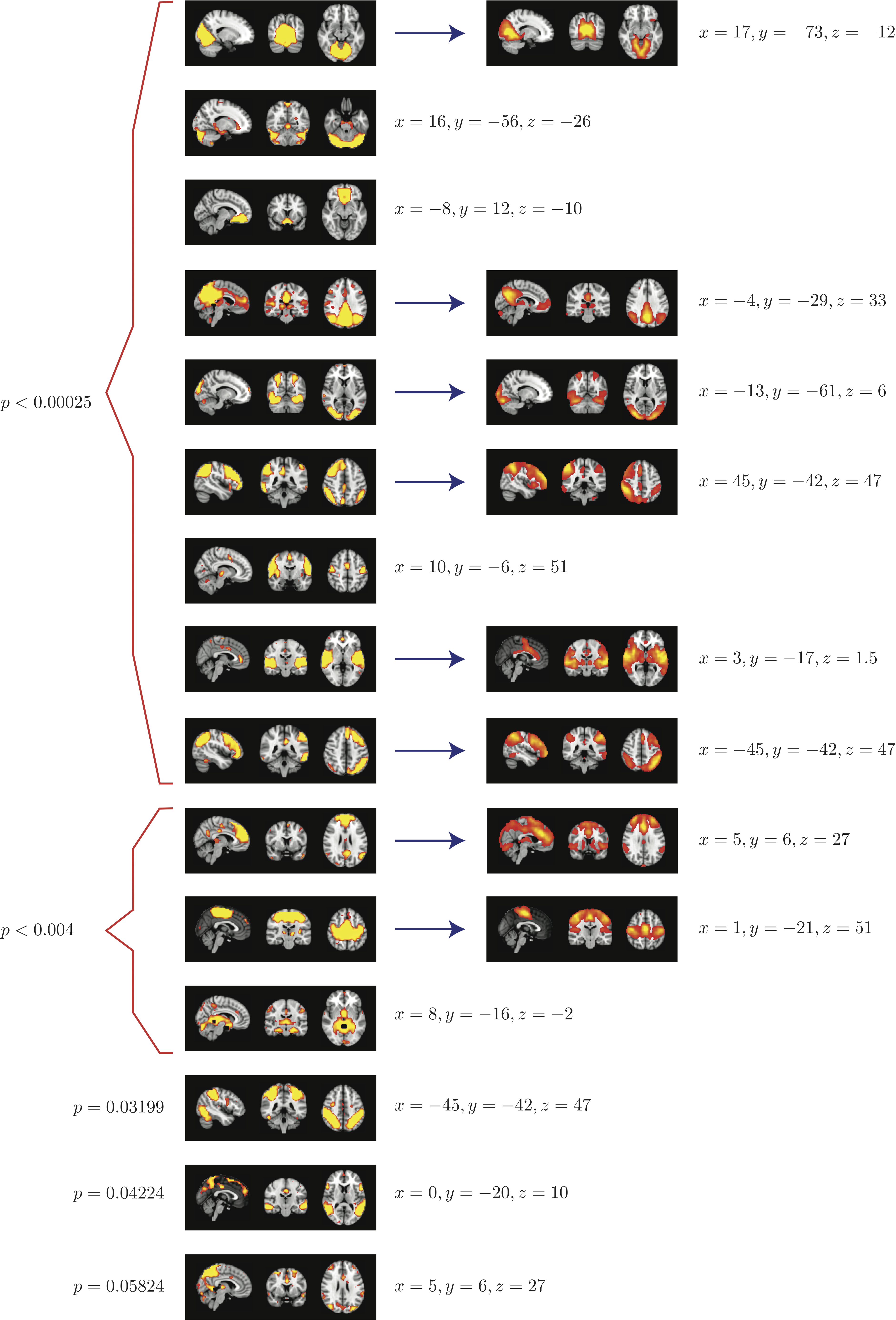3. ##### Summary
When 100 random 5 subject group ICA runs (from a population of 23 subjects) are combined using RAICAR_N:

• We are able to declare 8 "standard" RSNs as significantly reproducible at a p-value < 0.05.

• There are 6 other "non-standard" RSNs that can be declared as significantly reproducible at a p-value < 0.05.

• There is 1 other "non-standard" RSN that achieves a p-value of 0.05824.

A standalone version of this webpage for offline browsing can be downloaded here: raicar_N_webpage.zipunder the terms of the license described below.